Reinforcement Learning Dynamics in Social Dilemmas

Journal of Artificial Societies and Social Simulation vol. 11, no. 2 1
<https://www.jasss.org/11/2/1.html>

Received: 21-Jan-2007    Accepted: 17-May-2007    Published: 31-Mar-2008###Abstract

In this paper we replicate and advance Macy and Flache's (2002; Proc. Natl. Acad. Sci. USA, 99, 7229–7236) work on the dynamics of reinforcement learning in 2×2 (2-player 2-strategy) social dilemmas. In particular, we provide further insight into the solution concepts that they describe, illustrate some recent analytical results on the dynamics of their model, and discuss the robustness of such results to occasional mistakes made by players in choosing their actions (i.e. trembling hands). It is shown here that the dynamics of their model are strongly dependent on the speed at which players learn. With high learning rates the system quickly reaches its asymptotic behaviour; on the other hand, when learning rates are low, two distinctively different transient regimes can be clearly observed. It is shown that the inclusion of small quantities of randomness in players' decisions can change the dynamics of the model dramatically.

Keywords:
Reinforcement Learning; Replication; Game Theory; Social Dilemmas; Agent-Based; Slow Learning

Supporting material:
On-line model
Source code used to create every figure
Interactive trajectory maps

###Introduction

1.1
In two recent papers, Macy and Flache (2002; Flache and Macy 2002) explored the dynamics observed in 2×2 (2-player 2-strategy) social dilemma games when these are played by artificial agents using a particular type of reinforcement learning algorithm. Macy and Flache's work was subsequently advanced by Izquierdo et al. (2007), who formalised the solution concepts Macy and Flache had discovered, and used them to characterise the dynamics of their model for any 2×2 game. The present paper is also a continuation of Macy and Flache's work: we replicate Macy and Flache's (2002) model, summarise and illustrate some of the theoretical results derived by Izquierdo et al. (2007) and discuss the robustness of such analytical results to the inclusion of small quantities of noise in the agents' behaviour —i.e. we model players who occasionally make mistakes in choosing their actions—.

1.2
The method we use to advance our understanding of Macy and Flache's model is a combination of computer simulation experiments and theoretical analysis. The following two subsections provide some background on social dilemmas and reinforcement learning respectively. The introduction ends with an outline of the paper.

#### Social dilemmas

1.3
Social dilemmas are social interactions where everyone enjoys the benefits of collective action, but any individual would gain even more without contributing to the common good (provided that the others do not follow her defection). The problem of how to promote cooperation in these situations without having to resort to central authority has been fascinating scientists from a broad range of disciplines for decades. Such widespread interest is not at all surprising since, as Dawes (1980) wrote, the fundamental tensions that generate social dilemmas are present in the three crucial problems of the modern world: resource depletion, pollution, and overpopulation. Furthermore social dilemmas are by no means exclusive to human interactions: in many social contexts, regardless of the nature of their component units, we find that individual interests lead to collectively undesirable outcomes for which there is a feasible alternative that every individual would prefer.

1.4
At the most elementary level, social dilemmas can be formalised as two-person games where each player can either cooperate or defect. For each player i, the payoff when they both cooperate (Ri, for Reward) is greater than the payoff obtained when they both defect (Pi, for Punishment); when one cooperates and the other defects, the cooperator obtains Si (Sucker), whereas the defector receives Ti (Temptation). Assuming no two payoffs are equal, the essence of a social dilemma is captured by the fact that both players prefer any outcome in which the opponent cooperates to any outcome in which the opponent defects (min(Ti, Ri) > max(Pi, Si)), but they both can find reasons to defect. In particular, the temptation to cheat (if Ti > Ri) or the fear of being cheated (if Si < Pi) can put cooperation at risk. There are three well-known social dilemma games: Chicken, Stag Hunt, and the Prisoner's Dilemma. In Chicken the problem is greed but not fear (Ti > Ri > Si > Pi ; i = 1, 2); in Stag Hunt, the problem is fear but not greed (Ri > Ti > Pi > Si ; i = 1, 2); and finally, both problems coincide in the paradigmatic Prisoner's Dilemma (Ti > Ri > Pi > Si ; i = 1, 2). Macy and Flache (2002) consider the symmetric versions of these three social dilemma games (Ti = T ; Ri = R ; Pi = P ; Si = S ; i = 1, 2), but all the results in this paper are valid for any 2×2 game.

#### Reinforcement learning

1.5
Macy and Flache (2002) study a variant of Bush and Mosteller's (1955) linear stochastic model of reinforcement learning; this variant is a particular type of a wider class of aspiration-based reinforcement learning models (Bendor, Mookherjee and Ray 2001a). Reinforcement learners interact with their environment and use their experience to choose or avoid certain actions based on their consequences. Actions that led to satisfactory outcomes (i.e. outcomes that met or exceeded aspirations) in the past tend to be repeated in the future, whereas choices that led to unsatisfactory experiences are avoided.

1.6
The empirical study of reinforcement learning dates back to Thorndike's animal experiments on instrumental learning at the end of the 19th century (Thorndike 1898). The results of these experiments were formalized in the well known 'Law of Effect', which is nowadays one of the most robust properties of learning in the experimental psychology literature:
Of several responses made to the same situation those which are accompanied or closely followed by satisfaction to the animal will, other things being equal, be more firmly connected with the situation, so that, when it recurs, they will be more likely to recur; those which are accompanied or closely followed by discomfort to the animal will, other things being equal, have their connections to the situation weakened, so that, when it recurs, they will be less likely to occur. The greater the satisfaction or discomfort, the greater the strengthening or weakening of the bond. (Thorndike 1911, p. 244)

1.7
Nowadays there is little doubt that reinforcement learning is an important aspect of much learning in most animal species. In strategic contexts in general, empirical evidence suggests that reinforcement learning is most plausible in animals with imperfect reasoning abilities or in human subjects who have no information beyond the payoff they receive and may not even be aware of the strategic nature of the situation (Duffy 2006; Camerer 2003; Bendor, Mookherjee and Ray 2001a; Roth and Erev 1995; Mookherjee and Sopher 1994). In the context of experimental game theory with human subjects, several authors have used simple models of reinforcement learning to successfully explain and predict behaviour in a wide range of games (McAllister 1991; Roth and Erev 1995; Mookherjee and Sopher 1994; Mookherjee and Sopher 1997; Chen and Tang 1998; Erev and Roth 1998; Erev, Bereby-Meyer and Roth 1999). None of those games, however, were social dilemma games where, as in the three 2×2 games presented above, players could easily coordinate and benefit from mutual cooperation. The performance of reinforcement learning models to explain human behaviour in games that facilitate reciprocation, like the Prisoner's Dilemma, had not been as successful as in other types of games (e.g. zero-sum games and games with unique mixed strategy equilibria) until recently (Erev and Roth 2001). Contrary to the predictions of most models of reinforcement learning used in experimental game theory, many people do learn to cooperate in the repeated Prisoner's Dilemma. Erev and Roth (2001) have recently shown that such a result does not reflect a limitation of the reinforcement learning approach but derives from the fact that previous models used to fit experimental data assumed that players can only learn over immediate actions (i.e. stage-game strategies) but not over a strategy set including repeated-game strategies (like e.g. tit-for-tat).

1.8
In any case, the collection of models used to understand experimental evidence from the laboratory is only a small sample of all the reinforcement learning models that have been studied. Theoretical work (Karandikar et al. 1998; Pazgal 1997; Kim 1999; Palomino and Vega-Redondo 1999; Bendor, Mookherjee and Ray 2001a; Bendor, Mookherjee and Ray 2001b) has shown that mutual cooperation is a common long-run outcome in the three social dilemma games explained above for a broad family of models of reinforcement learning over immediate actions; furthermore, in certain settings it is the unique long-term outcome. The theoretical implications of aspiration-based reinforcement learning in strategic contexts have been studied thoroughly by Karandikar et al. (1998) and Bendor, Mookherjee and Ray (2001b). This theoretical line of work has focused on the long-run behaviour of reinforcement models in 2-player repeated games, for which sharp predictions for a wide range of reinforcement rules are now available (see Bendor, Mookherjee and Ray 2001a for an excellent overview). Importantly, the models analysed by this theoretical work assume players have a positive bias in favour of the most recently selected action —a feature called inertia (Bendor, Mookherjee and Ray 2001a; Bendor, Mookherjee and Ray 2001b)—. In contrast, the model that Macy and Flache (2002) investigate lacks inertia, so the mentioned theoretical results cannot be applied. A special case of Macy and Flache's model where all stimuli are necessarily positive was originally considered by Cross (1973) and analysed by Börgers and Sarin (1997), who showed the relation between this model and the replicator dynamics (Weibull 1995). This work was subsequently advanced by Izquierdo et al. (2007) who —using the theory of distance-diminishing models (Norman 1968, 1972)— provide theoretical results for the general case, where negative stimuli are also possible.

#### Outline of the paper

1.9
The rest of the paper is structured as follows: Section 2 describes the variant of Bush and Mosteller's (1955) linear stochastic model of reinforcement learning that Macy and Flache (2002) investigated. In section 3, following Izquierdo et al. (2007), we formalise Macy and Flache's concepts of dynamic equilibria (i.e. self-reinforcing equilibrium (SRE) and self-correcting equilibrium (SCE)). These concepts are not specific to their particular model; Flache and Macy (2002) demonstrate their generality using their General Reinforcement Learning model. Section 4 is included to familiarise the reader with the complex dynamics of the model under investigation. These dynamics are fully characterised in section 5 using the definitions of SRE and SCE. We then analyse the effect of including small quantities of noise in the model, and we finish with the conclusions.

1.10
The supporting material includes the Mathematica source code used to create every figure in the paper. For illustration and clarification purposes, we have also included an applet that can be used to replicate all the experiments presented here, and an interactive trajectory map.

###BM: An Agent-based Model of Reinforcement Learning

2.1
Macy and Flache (2002) used an elaboration of a conventional Bush-Mosteller (1955) stochastic learning model for binary choice; hence their model was named BM. In this model, players decide stochastically whether to cooperate or defect. Each player's strategy is defined by the probability of undertaking each of the two actions available to them. After every player has selected an action according to their probabilities, every player receives the corresponding payoff and revises her strategy. The revision of strategies takes place following a reinforcement learning approach: players increase their probability of undertaking a certain action if it led to payoffs above their aspiration level, and decrease this probability otherwise. When learning, players in the BM model use only information concerning their own past choices and payoffs, and ignore all the information regarding the payoffs and choices of their counterparts. More precisely, the updating of a strategy takes place in two steps. First, each player i calculates her stimulus sai for the action just chosen a (either Cooperate (C) or Defect (D)), according to the following formula (where every variable, including payoffs, is indexed in i):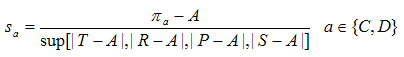where πa is the payoff obtained having selected action a, A is the player's aspiration level, and T , R , P , S are the possible payoffs the player might receive, as explained above. Hence the stimulus is always a number in the interval [-1, 1]. Secondly, having calculated their stimulus sai , each player i updates her probability pai of undertaking the selected action a as follows (where every variable is indexed in i):where pa,n is the probability of undertaking action a in time-step n, sa,n is the stimulus experienced after having selected action a in time-step n, and l is the learning rate (0 < l < 1). Thus the higher the stimulus (or the learning rate), the larger the change in probability. The updated probability for the action not selected derives from the constraint that probabilities must add up to one.

2.2
It is therefore clear that the state of the game can be fully characterized by a two-dimensional vector p = [ p1 , p2 ], where pi is player i's probability to cooperate. We will refer to such vector p as a strategy profile, or a state of the system. In the general case, a 2×2 BM model parameterisation requires specifying both players' payoffs (Ti , Ri , Pi , Si), aspiration level (Ai), and learning rate (li). Macy and Flache (2002) study systems where both players are parameterised in exactly the same way (homogeneous models), whereas our analysis is based on the results of Izquierdo et al. (2007), which are valid for any 2×2 game. A certain parameterization of a homogeneous model will be specified using the template [ T , R , P , S | A | l ]2. Homogeneous models will be used here for illustrative purposes, but all the results in this paper apply in the general case. On the other hand, Macy and Flache (2002) also consider models where aspiration levels may vary; in this paper we only study the case where aspiration levels are fixed.

2.3
The following notation will be useful: A parameterised model will be denoted S, for System. Let Pn(S) be the state of a system S in time-step n. Note that Pn(S) is a random variable and p is a particular value of that variable. Note also that the sequence of random variables {Pn(S)}n≥0 constitutes a discrete-time Markov process with an infinite number of (potentially transient) states.

###Attractors in the Dynamics of the System

3.1
Using the homogeneous BM model, Macy and Flache (2002) describe two types of attractors that govern the dynamics of their simulations: self-reinforcing equilibria (SRE) and self-correcting equilibria (SCE). These two concepts are not equilibria in the static sense of the word, but strategy profiles which act as attractors that pull the dynamics of the simulation towards them. Here, we formalize these two concepts.

3.2
Following Izquierdo et al. (2007), we define an SRE as an absorbing state of the system (i.e. a state p that cannot be abandoned) where both players receive a positive stimulus. An SRE corresponds to a pair of pure strategies (pi is either 0 or 1) such that its certain associated outcome gives a strictly positive stimulus to both players (henceforth a mutually satisfactory outcome). For example, the strategy profile [ 1 , 1 ] is an SRE if both players' aspiration levels are below their respective Ri. Escape from an SRE is impossible since no player will change her strategy. More importantly, SREs act as attractors: near an SRE, there is a high chance that the system will move towards it, because there is a high probability that its associated mutually satisfactory outcome will occur, and this brings the system even closer to the SRE. The number of SREs in a system is the number of outcomes where both players obtain payoffs above their respective aspiration levels.

3.3
Flache and Macy (2002, p. 634) define SCEs in the following way: "The SCE obtains when the expected change of probabilities is zero and there is a positive probability of punishment as well as reward". In this context, punishment means negative stimulus while reward means positive stimulus; the expected change of probability for one player is defined as the sum of the possible changes in probability the player might experience weighted by the likelihood of such changes actually happening. As we show below, SCEs defined in this way are not necessarily attractors, but may be unstable saddle points where small perturbations can cause expected probabilities to move away from them. Figure 1 represents the expected movement after one time-step for different states of the system in a Stag Hunt game. The Expected Motion (EM) of a system S in state p for the following iteration is given by a function vector EMS(p) whose components are the expected change in the probabilities to cooperate for each player. Mathematically,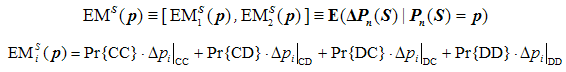where {CC, CD, DC, DD} represent the four possible outcomes that may occur. Note that in general the expected change will not reflect the actual change in a simulation run, and to make this explicit we have included the trace of a simulation run starting in state [ 0.5 , 0.5 ] in figure 1. The expected change —represented by the arrows in figure 1— is calculated considering the four possible changes that could occur (see equation above), whereas the actual change in a simulation run —represented by the numbered balls in figure 1— is only one of the four possible changes (e.g. Δpi|CC, if both agents happen to cooperate).Figure 1. Expected motion of the system in a Stag Hunt game parameterised as [ 3 , 4 , 1 , 0 | 0.5 | 0.5 ]2, together with a sample simulation run (40 iterations). The arrows represent the expected motion for various states of the system; the numbered balls show the state of the system after the indicated number of iterations in the sample run. The background is coloured using the norm of the expected motion. For any other learning rate the size of the arrows would vary but their direction would be preserved. The source code used to create this figure is available in the Supporting Material.

3.4
The state [ 0.5 , 0.5 ] in Figure 1 is an example of a strategy profile that satisfies Flache and Macy's requirements for SCE, but where small deviations tend to lead the system away from it (saddle point). To avoid such undesirable situations where an SCE is not self-correcting, Izquierdo et al. (2007) redefine the concept of SCE in a more restrictive way: an SCE of a system S is an asymptotically stable critical point (Mohler 1991) of differential equation  (the continuous time limit approximation of the system's expected motion).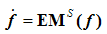(1)

or, equivalently,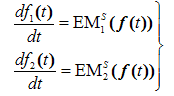3.5
Roughly speaking this means that all trajectories in the phase plane of Eq.  that at some instant are sufficiently close to the SCE will approach the SCE as the parameter t (time) approaches infinity and remain close to it at all future times. Note that, with this definition, there could be a state of the system that is an SRE and an SCE at the same time.

3.6
Figure 2 shows several trajectories for the differential equation corresponding to the Stag Hunt game used in Figure 1. It can be clearly seen that state [0.5 , 0.5] is not an SCE according to Izquierdo et al.'s definition, since there are trajectories that get arbitrarily close to it, but they then escape from its neighbourhood.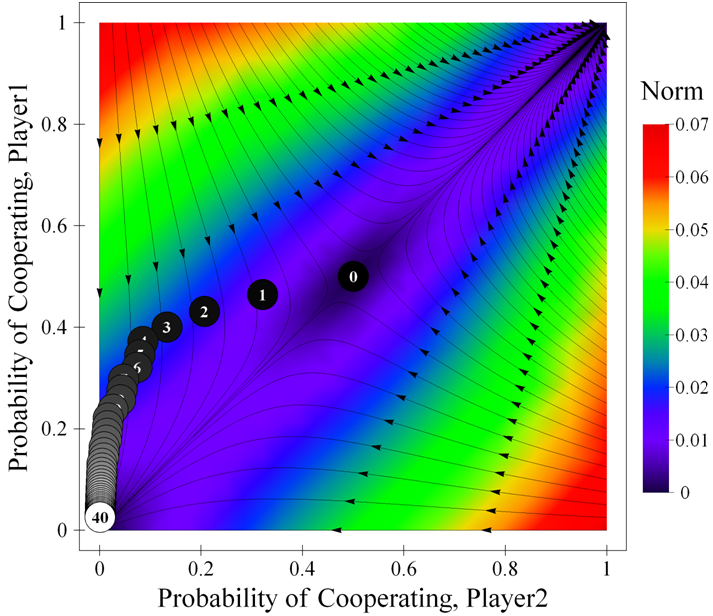Figure 2. Trajectories in the phase plane of the differential equation corresponding to a Stag Hunt game parameterised as [ 3 , 4 , 1 , 0 | 0.5 | 0.5 ]2, together with a sample simulation run (40 iterations). The background is coloured using the norm of the expected motion. The source code used to create this figure is available in the Supporting Material.

3.7
Figure 3 shows some trajectories of the differential equation corresponding to the Prisoner's Dilemma parameterised as [ 4 , 3 , 1 , 0 | 2 | l ]2. This system exhibits a unique SCE at [ 0.37 , 0.37 ] and a unique SRE at [ 1 , 1 ]. The function EM(p) for this system is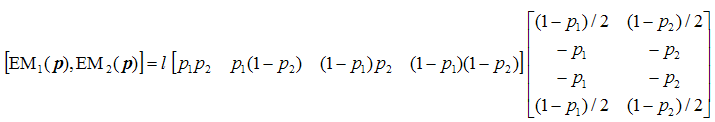And the associated differential equation is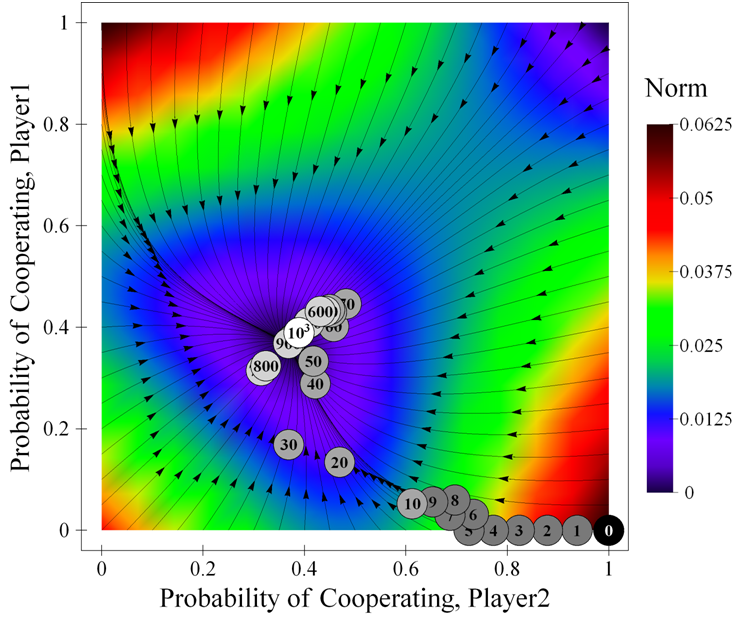Figure 3. Trajectories in the phase plane of the differential equation corresponding to the Prisoner's Dilemma game parameterised as [ 4 , 3 , 1 , 0 | 2 | l ]2, together with a sample simulation run ( l = 2-4 ). This system has an SCE at [ 0.37 , 0.37 ]. The background is coloured using the norm of the expected motion. The source code used to create this figure is available in the Supporting Material.

3.8
The expected motion at any point p in the phase plane is a vector tangent to the unique trajectory to which that point belongs. The use of expected motion (or mean-field) approximations to understand simulation models and to design interesting experiments has already proven to be very useful in the literature (e.g. Huet et al. 2007; Galán and Izquierdo 2005; Edwards et al. 2003; Castellano, Marsili, and Vespignani 2000). Note, however, that such approaches are approximations whose validity may be constrained to specific conditions: as we can see in Figure 3, simulation runs and trajectories will not coincide in general. In this paper we show that trajectories and SCEs are especially relevant for the transient dynamics of the system, particularly with small learning rates. On the other hand, we also show that the mean-field approximation can be misleading when studying the asymptotic behaviour of the model. From now on we will use Izquierdo et al.'s definitions of SRE and SCE.

###Complex Dynamics

4.1
The work reported in this paper originated after observing a puzzling phenomenon in Macy and Flache's experiments with the BM model. A significant part of their analysis consisted in studying for various parameter settings the proportion of simulation runs that "locked" into mutual cooperation. Such "lock-in rates" were as high as 1 in some experiments. However, in Macy and Flache's experiments, the BM model specifications guarantee that after any finite number of iterations any outcome has a positive probability of occurring. To investigate this apparent contradiction we conducted some qualitative analyses that we present here to familiarise the reader with the complex dynamics of this model. Our first qualitative analysis consisted in studying the expected dynamics of the model. Figure 4 illustrates the expected motion of a system extensively studied by Macy and Flache: the Prisoner's Dilemma game parameterised as [ 4 , 3 , 1 , 0 | 2 | 0.5 ]2. As we saw before, this system features a unique SCE at [ 0.37 , 0.37 ] and a unique SRE at [ 1 , 1 ]. Figure 4 also includes the trace of a sample simulation run.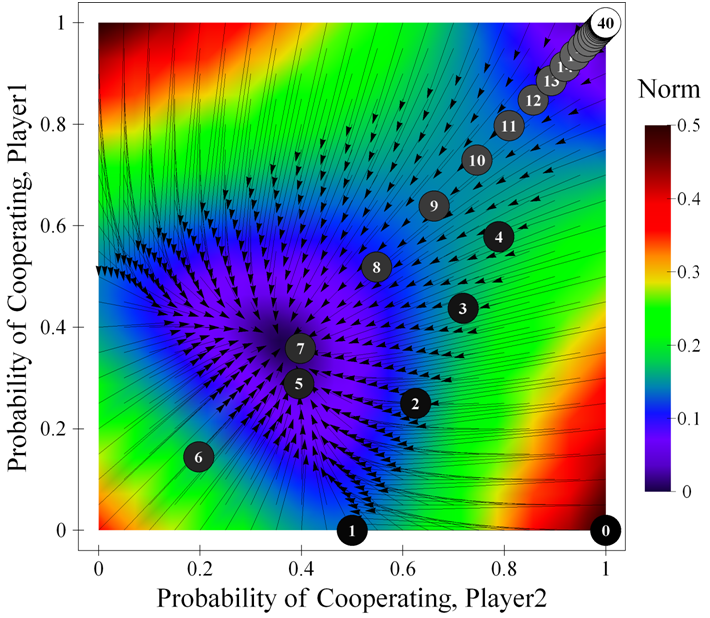Figure 4. Expected motion of the system in a Prisoner's Dilemma game parameterised as [ 4 , 3 , 1 , 0 | 2 | 0.5 ]2, with a sample simulation run. The background is coloured using the norm of the expected motion. The source code used to create this figure is available in the Supporting Material.

4.2
Figure 4 shows that the expected movement from any state is towards the SCE, except for the only SRE, which is an absorbing state. In particular, near the SRE, where both probabilities are high but different from 1, the distribution of possible movements is very peculiar: there is a very high chance that both agents will cooperate and consequently move a small distance towards the SRE, but there is also a positive chance, tiny as it may be, that one of the agents will defect, causing both agents to jump away from the SRE towards the SCE. The improbable —yet possible— leap away from the SRE is of such magnitude that the resulting expected movement is biased towards the SCE despite the unlikelihood of such an event actually occurring. The dynamics of the system can be further explored analysing the most likely movement from any given state, which is represented in Figure 5.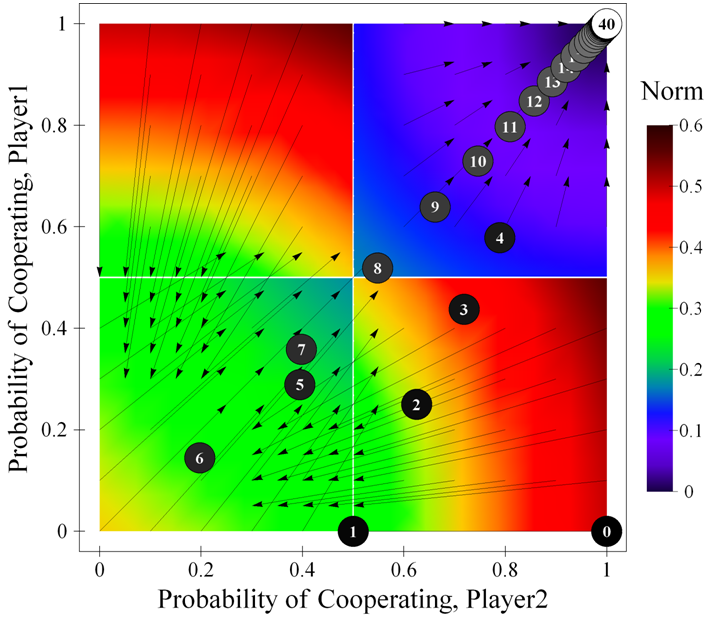Figure 5. Figure showing the most likely movements at some states of the system in a Prisoner's Dilemma game parameterised as [ 4 , 3 , 1 , 0 | 2 | 0.5 ]2, with a sample simulation run. The background is coloured using the norm of the expected motion. The source code used to create this figure is available in the Supporting Material.

4.3
Figure 5 differs significantly from Figure 4; it shows that the most likely movement in the upper-right quadrant of the state space is towards the SRE. Thus the walk towards the SRE is characterized by a fascinating puzzle: on the one hand, the most likely movement leads the system towards the SRE, which is even more likely to be approached the closer we get to it; on the other hand, the SRE cannot be reached in any finite number of steps and the expected movement as defined above is to walk away from it.

4.4
It is also interesting to note in this game that, starting from any mixed (interior) state, both players have a positive probability of selecting action D in any future time-step, but there is also a positive probability of both players engaging in an infinite chain of the mutually satisfactory event CC forever, i.e., that neither player will ever take action D from then onwards. This latter probability can be calculated using a result that we present in the Appendix.

4.5
The probability of starting an infinite chain of CC events depends largely on the value of the learning rate l. Figure 6 shows the probability of starting an infinite chain of the mutually satisfactory outcome CC in a Prisoner's Dilemma game parameterised as [ 4 , 3 , 1 , 0 | 2 | l ]2, for different learning rates l, and different initial probabilities to cooperate x0 (the same probability for both players). For some values, the probability of immediately starting an infinite chain of mutual cooperation can be surprisingly high (e.g. for l = 0.5 and initial conditions [ x0 , x0 ] = [ 0.9 , 0.9 ] such probability is approximately 44%).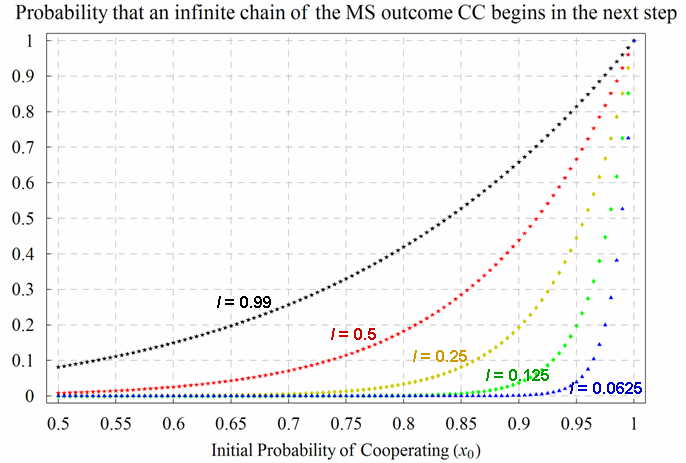Figure 6. Probability of starting an infinite chain of the Mutually Satisfactory (MS) outcome CC in a Prisoner's Dilemma game parameterised as [ 4 , 3 , 1 , 0 | 2 | l ]2. The 5 different (coloured) series correspond to different learning rates l. The variable x0, represented in the horizontal axis, is the initial probability of cooperating for both players. The source code used to create this figure is available in the Supporting Material.

###Different Regimes in the Dynamics of the System

5.1
This section illustrates the dynamics of the BM model for different learning rates. The analysis is presented here in a somewhat qualitative fashion for the sake of clarity and comprehensibility, and illustrates the behaviour of the model that we are replicating in some social dilemmas. Most of the theoretical results that we apply and summarise in this section are valid for any 2×2 game and can be found in Izquierdo et al. (2007).

5.2
In the general case, the dynamics of the BM model may exhibit three different regimes: medium run, long run, and ultralong run. The terminology we use here is borrowed from Binmore and Samuelson (1993) and Binmore, Samuelson and Vaughan (1995), who reserve the term short run for the initial conditions.
By the ultralong run, we mean a period of time long enough for the asymptotic distribution to be a good description of the behavior of the system. The long run refers to the time span needed for the system to reach the vicinity of the first equilibrium in whose neighborhood it will linger for some time. We speak of the medium run as the time intermediate between the short run [i.e. initial conditions] and the long run, during which the adjustment to equilibrium is occurring. (Binmore, Samuelson and Vaughan 1995, p. 10)

5.3
Binmore et al.'s terminology is particularly useful for our analysis because it is often the case in the BM model that the long run, which is characterised by the "first equilibrium in whose neighborhood it [the system] will linger for some time" is significantly different from the asymptotic dynamics of the system, i.e. the ultralong run. Whether the three different regimes are clearly distinguishable in the BM model strongly depends on the players' learning rates. For high learning rates the system quickly approaches its asymptotic behaviour (the ultralong run) and the distinction between the different regimes is not particularly useful. For small learning rates, however, the three different regimes can be clearly observed. Since the ultralong run is the only regime that (sooner or later) is observed in every system, we start our description of the dynamics of the BM model characterising such regime.

5.4
Izquierdo et al. (2007) prove that most BM systems —in particular all the systems studied by Macy and Flace (2002) with fixed aspirations— converge to an SRE if there exits at least one SRE, confirming an insight already provided by Macy and Flache (2002) and Flache and Macy (2002). The probability of the process converging to one particular SRE depends on the initial state. If the initial state is completely mixed, then every SRE can be (asymptotically) reached with positive probability. If there are no SREs, the system converges to a distribution which is independent of the initial conditions. In the context of the social dilemma games described above, this implies that if players' aspirations are above their respective maximin, then the ultralong run is independent of the initial state. Under such conditions, there is an SRE if and only if mutual cooperation is satisfactory for both players and, if that is the case, the process converges to certain mutual cooperation (i.e. the unique SRE) with probability 1. As an example, note that the asymptotic behaviour of the systems shown in figures 3, 4 and 5 is certain mutual cooperation.

#### Learning by Large Steps (Fast Adaptation)

5.5
As mentioned above, when learning takes place by large steps, the system quickly reaches its ultralong run behaviour. To explain why this is the case we distinguish between two possible classes of systems:
• In systems where there is at least one SRE, the asymptotic behaviour is quickly approached because SREs are powerful attractors. The reason for this is that, if an SRE exists, the chances of a mutually satisfactory outcome not occurring for a long time are low, since players update their strategies to a large extent to avoid unsatisfactory outcomes. Whenever a mutually satisfactory outcome occurs, players update their strategy so the chances of repeating such a mutually satisfactory outcome increase. Since learning rates are high, the movement towards the SRE associated with such a mutually satisfactory outcome takes place by large steps, so only a few coordinated moves are sufficient to approach the SRE so much that escape from its neighbourhood becomes very unlikely. In other words, with fast learning the system quickly approaches an SRE, and is likely to keep approaching that SRE forever. As an example, consider Figure 6 again: starting from any initial probability to cooperate x0, the occurrence of a mutually satisfactory outcome CC would increase both players' probability to cooperate (the updated probability can be seen as the following period's x0), which in turn would increase the probability of never defecting (i.e., the probability of starting an infinite chain of CC). Thus, if the learning rate is large, a few CC events are enough to take the state of the system into areas where the probability of never defecting again is large.
• In the absence of SREs, the fact that any outcome is unsatisfactory for at least one of the players and the fact that strategy changes are substantial, together imply that at least one player will switch between actions very frequently —i.e. the system will indefinitely move rapidly and widely around a large area of the state space—.

#### Learning by Small Steps (Slow Adaptation)

5.6
Flache and Macy (2002), and Macy and Flache (2002), referring to earlier results by Macy (1989; 1991), show how lowering learning rates increases the time that the system spends close to the SCE before leaving for the SRE. Here, following theoretical results by Izquierdo et al. (2007, Proposition 1) we show how:
• for low enough learning rates, the BM process tends to follow a specific trajectory in the phase plane of Eq.  (which trajectory in particular depends on the initial conditions).
• for low enough learning rates, the BM process in time-step n tends to be concentrated around a particular point in the trajectory (this point depends on the particular values of n and l).
• if trajectories get close to an SCE (as t grows), then, for low learning rates, the BM process will tend to approach and linger around the SCE; the lower the learning rate, the greater the number of periods that the process will tend to stay around the SCE.

5.7
When learning takes place by small steps the other two regimes (i.e. the medium and the long run) can be clearly observed, and these transient dynamics can be substantially different from the ultralong run behaviour of the system. For sufficiently small learning rates and number of iterations n not too large (n·l bounded), the medium run dynamics of the system are best characterised by the trajectories in the phase plane of Eq. , which can follow paths substantially apart from the end-states of the system (see figure 7, where the end-state is [1 , 1]). Under such conditions, the expected state of the system after n iterations can be estimated by substituting the value n·l in the trajectory that commences at the initial conditions. The lower the learning rates, the better the estimate, i.e. the more tightly clustered the dynamics will be around the corresponding trajectory in the phase plane (see figure 7).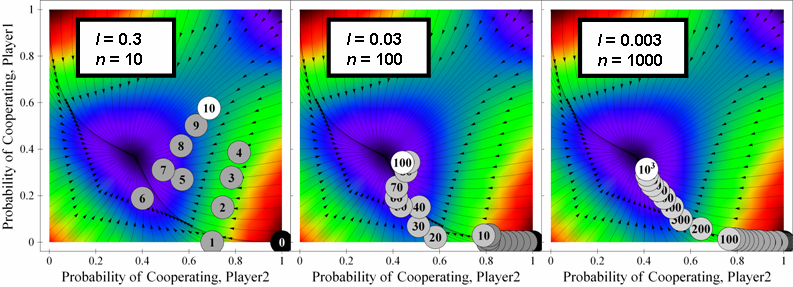Figure 7. Three sample runs of a system parameterised as [ 4 , 3 , 1 , 0 | 2 | l ]2. for different values of n and l. The product n·l is the same for the three simulations; therefore, for low values of l, the state of the system at the end of the simulations tends to concentrate around the same point. The source code used to create this figure is available in the Supporting Material.

5.8
When trajectories finish in an SCE, the system will approach the SCE and spend a significant amount of time in its neighbourhood if learning rates are low enough and the number of iterations n is large enough (and finite). This latter regime is the long run. The fact that trajectories are good approximations for the transient dynamics of the system for slow learning shows the importance of SCEs —points that "attract" trajectories within their neighbourhood— as attractors of the actual dynamics of the system. This is particularly so when, as in most 2×2 games, there are very few asymptotically stable critical points and they have very wide domains of attraction.

5.9
Remember, however, that the system will eventually approach its asymptotic behaviour, which in the systems shown in figures 3, 4, 5, 6 and 7 is certain mutual cooperation. Having said that, as Binmore, Samuelson and Vaughan (1995) point out, the length of time required for the asymptotic distribution to be relevant may be extraordinarily long, much longer than is often meant by long run, hence the term ultralong run.

5.10
To illustrate how learning rates affect the speed of convergence to asymptotic behaviour, consider once again the Prisoner's Dilemma game parameterised as [ 4 , 3 , 1 , 0 | 2 | l ]2, a system extensively studied by Macy and Flache (2002). The evolution of the probability to cooperate (which is identical for both players) for two learning rates l is represented in Figure 8. The top row shows the evolution for l = 0.5 (figures 4 and 5 show a sample run of this system), and the bottom row shows the evolution for l = 2-4 (figure 3 shows a sample run of this system). For l = 0.5, after only 29 = 512 iterations, the probability that both players will be almost certain to cooperate is very close to 1, and it remains so thereafter. For l = 2-4, however, the distribution is still clustered around the SCE even after 221 = 2097152 iterations. In the latter case, the chain of events that is required to escape from the neighbourhood of the SCE is extremely unlikely, and therefore this long run regime seems to persist indefinitely. However, given sufficient time, such a chain of coordinated moves will occur, and the system will eventually reach its ultralong run regime, i.e. almost-certain mutual cooperation.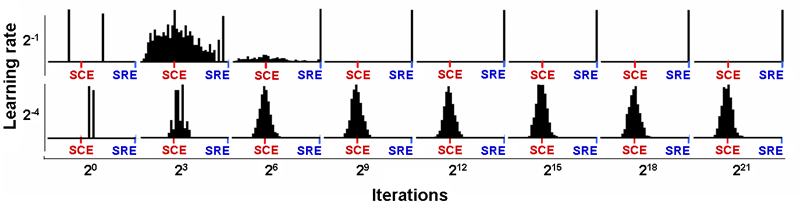Figure 8. Histograms representing the probability of cooperating for one player (both players' probabilities are identical) after n iterations for different learning rates l in a Prisoner's Dilemma game parameterised as [ 4 , 3 , 1 , 0 | 2 | l ]2, each calculated over 1,000 simulation runs. The initial probability for both players is 0.5. The source code used to create this figure is available in the Supporting Material.

###Trembling hands process

6.1
To study the robustness of the previous asymptotic results we consider an extension of the BM model where players suffer from "trembling hands" (Selten 1975): after having decided which action to undertake, each player i may select the wrong action with some probability εi > 0 in each iteration. This noisy feature generates a new stochastic process, namely the noisy process Nn, which can also be fully characterized by a 2-dimensional vector prop = [prop1 , prop2 ] of propensities (rather than probabilities) to cooperate. Player i's actual probability to cooperate is now (1 – εi) · propi + εi · (1 – propi), and the profile of propensities prop evolves after any particular outcome following the rules given in section 2. Izquierdo et al. (2007) prove that the noisy process Nn is ergodic in any 2×2 game. Ergodicity implies that the state of the process presents an asymptotic probability distribution which does not depend on the initial state.

6.2
The noisy process has no absorbing states (i.e. SREs) except in the trivial case where both players find one of their actions always satisfactory and the other action always unsatisfactory —thus, for example, in the three 2×2 social dilemma games the inclusion of noise precludes the system from convergence to a single state—. However, even though noisy processes have no SREs in general, the SREs of the associated unperturbed process (SREUPs, which correspond to mutually satisfactory outcomes) do still act as attractors whose attractive power depends on the magnitude of the noise: ceteris paribus the lower the noise the higher the long run chances of finding the system in the neighborhood of an SREUP (see Figure 9). This is so because in the proximity of an SREUP, if εi are low enough, the SREUP's associated mutually satisfactory outcome will probably occur, and this brings the system even closer to the SREUP. The dynamics of the noisy system will generally be governed also by the other type of attractor, the SCE.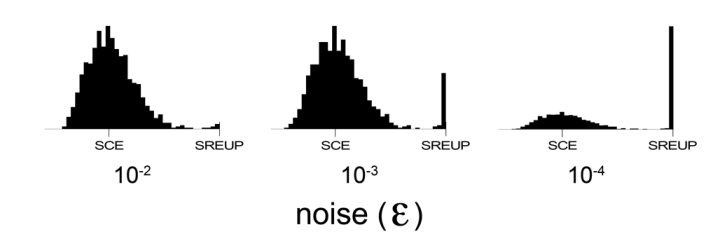Figure 9. Histograms representing the propensity to cooperate for one player (both players' propensities are identical) after 1,000,000 iterations (when the distribution is stable) for different levels of noise (εi = ε) in a Prisoner's Dilemma game parameterised as [ 4 , 3 , 1 , 0 | 2 | 0.25 ]2. Each histogram has been calculated over 1,000 simulation runs. The source code used to create this figure is available in the Supporting Material.

6.3
Figures 10, 11 and 12, which correspond to a Prisoner's Dilemma game parameterised as [ 4 , 3 , 1 , 0 | 2 | l ]2, show that the presence of noise can greatly damage the stability of the (unique) SREUP associated to the event CC. Note that the inclusion of noise implies that the probability of an infinite chain of the mutually satisfactory event CC becomes zero.

6.4
In Figure 10, corresponding to a learning rate l = 0.5, the system shows a tendency to be quickly attracted towards the SRE, but the presence of noise breaks (from time to time) the chains of mutually satisfactory CC events; unilateral defections make the system escape from the area of the SREUP before going back towards it again, and so forth.

6.5
In Figure 11, corresponding to a lower learning rate (l = 0.25) than in Figure 10, the system shows a tendency to be lingering around the SCE for longer. In this case, when a unilateral defection breaks a chain of mutually satisfactory events CC and the system leaves the proximity of the SREUP, it usually takes a large number of periods to go back into that area.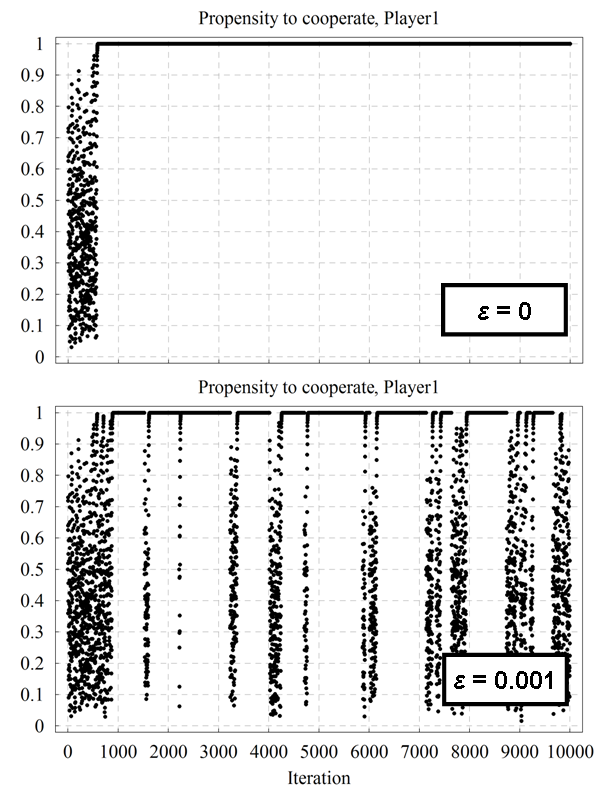Figure 10. A representative time series of player 1's propensity to cooperate over time for the Prisoner's Dilemma game parameterised as [ 4 , 3 , 1 , 0 | 2 | 0.5 ]2 with initial conditions [ x0 , x0 ] = [ 0.5 , 0.5 ], both without noise (top) and with a noise level εi = 10-3 (bottom). The source code used to create this figure is available in the Supporting Material.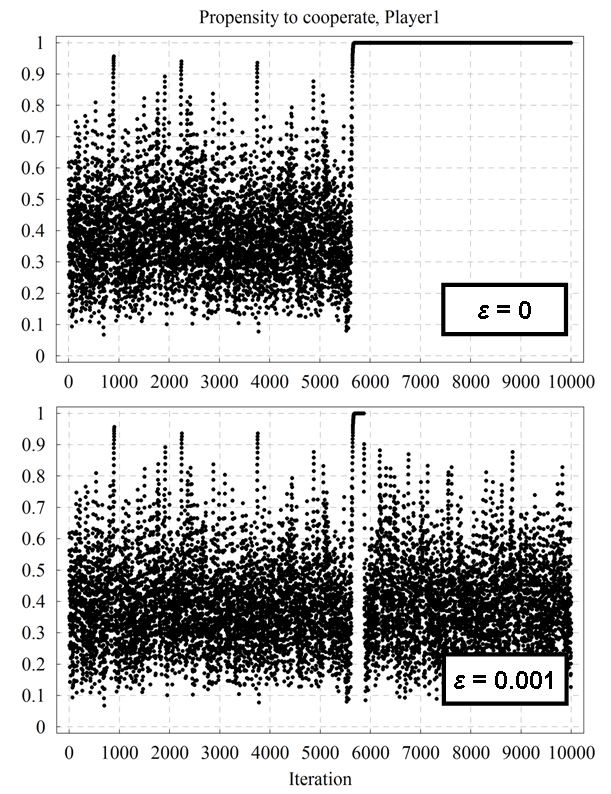Figure 11. A representative time series of player 1's propensity to cooperate over time for the Prisoner's Dilemma game parameterised as [ 4 , 3 , 1 , 0 | 2 | 0.25 ]2 with initial conditions [ x0 , x0 ] = [ 0.5 , 0.5 ], both without noise (top) and with a noise level εi = 10-3 (bottom). The source code used to create this figure is available in the Supporting Material.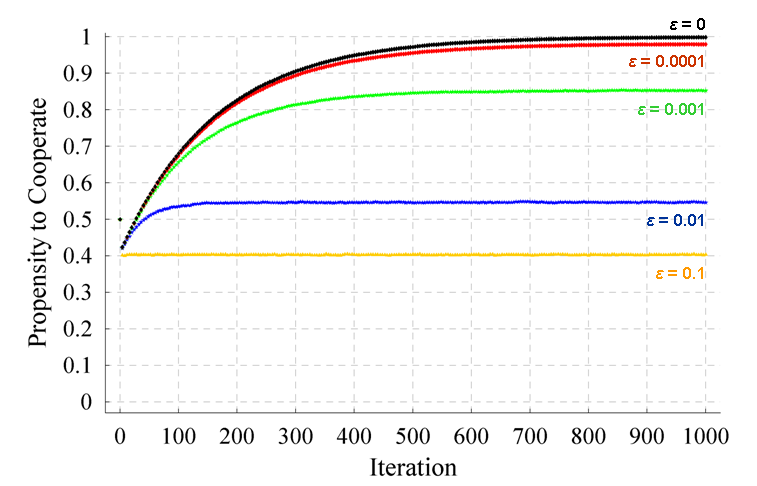Figure 12. Evolution of the average probability / propensity to cooperate of one of the players in a Prisoner's Dilemma game parameterised as [ 4 , 3 , 1 , 0 | 2 | 0.5 ]2 with initial state [ 0.5 , 0.5 ], for different levels of noise (εi = ε). Each series has been calculated averaging over 100,000 simulation runs. The standard error of the represented averages is lower than 3·10-3 in every case. The source code used to create this figure is available in the Supporting Material.

6.6
As shown in Figure 12, the greater the noise level, the higher the destabilisation of the SREUP. This is so because, even in the proximity of the SREUP, the long chains of reinforced CC events that stabilise the SREUP become highly unlikely when there are high levels of noise, and unilateral defections (whose probability grows with the noise level in the proximity of the SREUP) break the stability of the SREUP.

#### Stochastic stability

6.7
Importantly, not all the SREs of the unperturbed process are equally robust to noise. As mentioned above, if there is any SRE, the unperturbed system converges to an SRE. The probability of the process converging to one particular SRE depends on the initial state, and if the initial state is completely mixed, then convergence to any of the SREs is possible (Izquierdo et al. 2007). Looking at the line labelled "ε = 0" in figure 13 we can see that the system [ 4 , 3 , 1 , 0 | 0.5 | 0.5 ]2 with initial state [ 0.9 , 0.9 ] has a probability of converging to its SRE at [ 1 , 1 ] approximately equal to 0.7, and a probability of converging to its SRE at [ 0 , 0 ] approximately equal to 0.3.

6.8
For low enough levels of "trembling hands" noise we find an asymptotic (invariant) distribution concentrated on neighbourhoods of SREUPs. The lower the noise, the higher the concentration around SREUPs. If there are several SREUPs, the invariant distribution may concentrate around some of these SREUPs much more than around others. In the limit as the noise goes to zero, it is often the case that only some of the SREUPs remain points of concentration. These are called stochastically stable equilibria (Foster and Young 1990; Young 1993; Ellison 2000). As an example, consider the simulation results shown in figure 13, which clearly suggest that the SRE at [ 0 , 0 ] is the only stochastically stable equilibrium even though, with initial conditions [ 0.9 , 0.9 ], the unperturbed process converges to the other SRE more frequently. Note that whether an equilibrium is stochastically stable or not is independent on the initial conditions.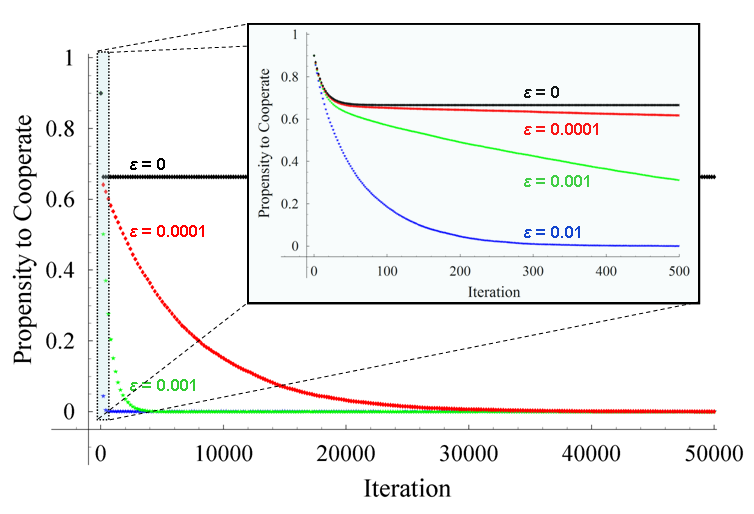Figure 13. Evolution of the average probability / propensity to cooperate of one of the players in a Prisoner's Dilemma game parameterised as [ 4 , 3 , 1 , 0 | 0.5 | 0.5 ]2 with initial state [ 0.9 , 0.9 ], for different levels of noise (εi = ε). Each series has been calculated averaging over 10,000 simulation runs. The inset graph is a magnification of the first 500 iterations. The standard error of the represented averages is lower than 0.01 in every case. The source code used to create this figure is available in the Supporting Material.

6.9
Intuitively, note that in the system shown in figure 13, in the proximities of the SRE at [ 1 , 1 ], one single (possibly mistaken) defection is enough to lead the system away from it. On the other hand, near the SRE at [ 0 , 0 ] one single (possibly mistaken) cooperation will make the system approach this SRE at [ 0 , 0 ] even more closely. Only a coordinated mutual cooperation (which is highly unlikely near the SRE at [ 0 , 0 ]) will make the system move away from this SRE. This makes the SRE at [ 0 , 0 ] much more robust to occasional mistakes made by the players when selecting their strategies, as illustrated in figures 14 and 15.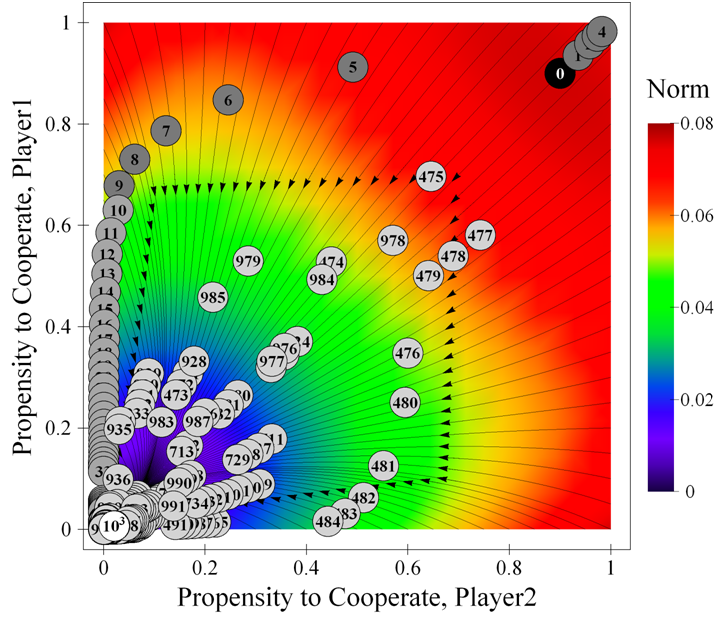Figure 14. One representative run of the system parameterised as [ 4 , 3 , 1 , 0 | 0.5 | 0.5 ]2 with initial state [ 0.9 , 0.9 ], and noise εi = ε = 0.1. This figure shows the evolution of the system in the phase plane of propensities to cooperate, while figure 15 below shows the evolution of player 1's propensity to cooperate over time for the same simulation run. The background is coloured using the norm of the expected motion. The source code used to create this figure is available in the Supporting Material.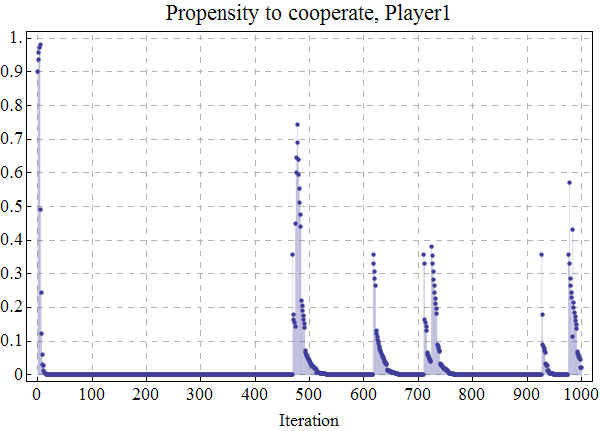Figure 15. Time series of player 1's propensity to cooperate over time for the same simulation run displayed in figure 14. The source code used to create this figure is available in the Supporting Material.

###Conclusions

7.1
In this paper we have replicated and advanced Macy and Flache's (2002; Flache and Macy 2002) work on the dynamics of a particular form of aspiration-based reinforcement learning in 2×2 social dilemmas. We have shown how their concepts of self-reinforcing equilibrium (SRE) and self-correcting equilibrium (SCE), as formalised by Izquierdo et al. (2007), can be meaningfully used to analyse the dynamics of their model. These dynamics are strongly dependent on the speed at which players learn. With high learning rates, it is shown that the model approaches its asymptotic behaviour fairly quickly. For most parameterisations of the model, such asymptotic dynamics are concentrated in the SREs of the system. On the other hand, with low learning rates, the dynamics of the system are likely to go through two transient distinct regimes before approaching the asymptotic regime. Such transient dynamics are strongly linked to the solutions of the continuous time limit approximation of the system's expected motion.

7.2
We have also shown that the inclusion of small quantities of noise in the model can change its dynamics dramatically. States of the system that are asymptotically reached with high probability in the unperturbed model may be observed with arbitrarily low probability when players make occasional mistakes in selecting their actions. Future work will be devoted to formally identifying the conditions under which asymptotic equilibria of the unperturbed process are robust to small trembles (i.e. characterising the set of stochastically stable equilibria).

###Acknowledgements

We are grateful to the Scottish Executive Environment and Rural Affairs Department and to the Spanish Ministry of Education and Science (Projects DPI2004-06590 and DPI2005-05676) for providing some financial support to conduct this piece of research. We are also very grateful to Jörgen W. Weibull for many helpful and insightful comments and for deriving the mathematical result used to produce figure 6, and to Alexandre Eudes, Alexis Revue and Vincent Barra, for helping to implement the applet provided in the Supporting Material.

###Notes

1 The aspiration level is a constant value for each player, and it is assumed to be different from every payoff the player may receive.

2 The concept of SRE is extensively used by Macy and Flache but we have not found a clear definition in their papers (Macy and Flache 2002; Flache and Macy 2002). Sometimes their use of the word SRE seems to follow our definition (e.g. Macy and Flache 2002, p. 7231), but often it seems to denote a mutually satisfactory outcome (e.g. Macy and Flache 2002, p. 7231) or an infinite sequence of such outcomes (e.g. Macy and Flache 2002, p. 7232).

3 The specification of the model is such that probabilities cannot reach the extreme values of 0 or 1 starting from any other intermediate value. Therefore if we find a simulation run that has actually ended up in the lock-in state [ 1 , 1 ] starting from any other state, we know for sure that such simulation run did not follow the specifications of the model (e.g. perhaps because of floating-point errors). For a detailed analysis of the effects of floating point errors in computer simulations, with applications to this model in particular, see Izquierdo and Polhill (2006), Polhill and Izquierdo (2005), Polhill et al. (2006), Polhill et al. (2005).

4 Maximin is the largest possible payoff players can guarantee themselves. In the three 2×2 social dilemmas maximini = max(Si, Pi).

5 Recall that each player's aspiration level is assumed to be different from every payoff the player may receive.

6 Excluded here is the trivial case where the initial state is an SRE.

7 We exclude here the meaningless case where the payoffs for some player are all the same and equal to her aspiration (Ti = Ri = Pi = Si = Ai for some i).

###Appendix

A.1
We provide here a theoretical result that can be used to estimate with arbitrary precision the probability L that an infinite sequence of a mutually satisfactory outcome mso = (a1 , a2), where player 1 selects action a1 and player 2 selects action a2, begins when the system is in state [p1 , p2], where pi denotes player i's probability to select action ai.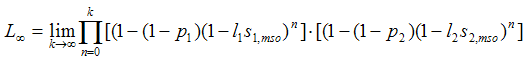where li denotes player i's learning rate, and si,mso denotes player i's stimulus after the mutually satisfactory outcome mso. The following result can be used to estimate L with arbitrary precision:

Let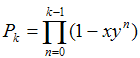and let.
Then, for x, y in the interval (0, 1),This result is based on the boundThe proof of this bound can be found in Bush and Mosteller (1955), who acknowledge assistance by William J. McGill. We are indebted to Professor Jörgen W. Weibull for his help with these calculations.

###Supporting Material

On-line model
Source code used to create every figure
Interactive trajectory maps

###References

BENDOR J, Mookherjee D and Ray D (2001a) Aspiration-Based Reinforcement Learning in Repeated Interaction Games: an Overview. International Game Theory Review 3(2-3), 159-174.

BENDOR J, Mookherjee D and Ray D (2001b) Reinforcement Learning in Repeated Interaction Games. Advances in Theoretical Economics 1(1), Article 3.

BINMORE K and Samuelson L (1993) An Economist's Perspective on the Evolution of Norms. Journal of Institutional and Theoretical Economics 150, 45-63.

BINMORE K, Samuelson L and Vaughan R (1995) Musical Chairs: Modeling Noisy Evolution. Games and Economic Behavior 11(1), 1-35.

BÖRGERS T and Sarin R (1997) Learning through Reinforcement and Replicator Dynamics. Journal of Economic Theory 77(1), 1-14.

BUSH R and Mosteller F (1955) Stochastic Models of Learning. John Wiliey & Son, New York.

CAMERER C F (2003) Behavioral Game Theory: Experiments in Strategic Interaction. Russell Sage Foundation, New York.

CASTELLANO C, Marsili M, and Vespignani A (2000) Nonequilibrium phase transition in a model for social influence. Physical Review Letters, 85(16), pp. 3536-3539.

CHEN Y and Tang F (1998) Learning and Incentive-Compatible Mechanisms for Public Goods Provision: An Experimental Study. Journal of Political Economy 106(3), 633-662.

CROSS J G (1973) A Stochastic Learning Model of Economic Behavior. Quartertly Journal of Economics 87(2), 239-266.

DAWES R M (1980) Social Dilemmas. Annual Review of Psychology 31, 169-93.

DUFFY J (2006) Agent-Based Models and Human Subject Experiments. In: Tesfatsion, L., Judd, K. L. (Eds.), Handbook of Computational Economics II: Agent-Based Computational Economics, chapter 19, 949-1011. Amsterdam: Elsevier.

EDWARDS M, Huet S, Goreaud F and Deffuant G (2003) Comparing an individual-based model of behaviour diffusion with its mean field aggregate approximation. Journal of Artificial Societies and Social Simulation 6(4)9. https://www.jasss.org/6/4/9.html.

ELLISON G (2000) Basins of Attraction, Long-Run Stochastic Stability, and the Speed of Step-by-Step Evolution. Review of Economic Studies 67, 17-45.

EREV I and Roth A E (1998) Predicting How People Play Games: Reinforcement Learning in Experimental Games with Unique, Mixed Strategy Equilibria. American Economic Review 88(4), 848-881.

EREV I, Bereby-Meyer Y and Roth A E (1999) The effect of adding a constant to all payoffs: experimental investigation, and implications for reinforcement learning models. Journal of Economic Behavior and Organization 39(1), 111-128.

EREV I and Roth A E (2001) Simple Reinforcement Learning Models and Reciprocation in the Prisoner's Dilemma Game. In: Gigerenzer, G., Selten, R. (Eds.), Bounded rationality: The Adaptive Toolbox. MIT Press, Cambridge.

FLACHE A and Macy M W (2002) Stochastic Collusion and the Power Law of Learning. Journal of Conflict Resolution 46(5), 629-653.

FOSTER D and Young H P (1990) Stochastic Evolutionary Game Dynamics. Theoretical Population Biology 38, 219-232.

GALÁN J M and Izquierdo L R (2005) Appearances Can Be Deceiving: Lessons Learned Re-Implementing Axelrod's 'Evolutionary Approach to Norms'. Journal of Artificial Societies and Social Simulation 8(3)2. https://www.jasss.org/8/3/2.html.

HUET S, Edwards M, and Deffuant G (2007) Taking into Account the Variations of Neighbourhood Sizes in the Mean-Field Approximation of the Threshold Model on a Random Network. Journal of Artificial Societies and Social Simulation 10(1)10. https://www.jasss.org/10/1/10.html.

IZQUIERDO L R, Polhill J G (2006). Is Your Model Susceptible to Floating-Point Errors?. Journal of Artificial Societies and Social Simulation 9(4)4. https://www.jasss.org/9/4/4.html.

IZQUIERDO L R, Izquierdo S S, Gotts N M and Polhill J G (2007) Transient and Asymptotic Dynamics of Reinforcement Learning in Games. Games and Economic Behavior 61(2), pp. 259-276.

KARANDIKAR R, Mookherjee D, Ray D and Vega-Redondo F (1998) Evolving Aspirations and Cooperation. Journal of Economic Theory 80(2), 292-331.

KIM Y (1999) Satisficing and optimality in 2×2 common interest games. Economic Theory 13(2), 365-375.

MACY M W (1989) Walking out of social traps: A stochastic learning model for the Prisoner's Dilemma. Rationality and Society 1(2), 197-219.

MACY M W (1991) Learning to cooperate: Stochastic and tacit collusion in social exchange. The American Journal of Sociology 97(3), 808-843.

MACY M W and Flache A (2002) Learning Dynamics in Social Dilemmas. Proceedings of the National Academy of Sciences USA 99(3), 7229-7236.

MCALLISTER P H (1991) Adaptive approaches to stochastic programming. Annals of Operations Research 30(1), 45-62.

MOHLER R R (1991) Nonlinear Systems, Volume I: Dynamics and Control. Prentice Hall, Englewood Cliffs.

MOOKHERJEE D and Sopher B (1994) Learning Behavior in an Experimental Matching Pennies Game. Games and Economic Behavior 7(1), 62-91.

MOOKHERJEE D and Sopher B (1997) Learning and Decision Costs in Experimental Constant Sum Games. Games and Economic Behavior 19(1), 97-132

NORMAN M F(1968) Some Convergence Theorems for Stochastic Learning Models with Distance Diminishing Operators. Journal of Mathematical Psychology 5(1), 61-101.

NORMAN M F(1972) Markov Processes and Learning Models. Academic Press, New York.

PALOMINO F and Vega-Redondo F (1999) Convergence of Aspirations and (partial) cooperation in the Prisoner's Dilemma. International Journal of Game Theory 28(4), 465-488.

PAZGAL A (1997) Satisficing Leads to Cooperation in Mutual Interests Games. International Journal of Game Theory 26(4), 439-453.

POLHILL J G and Izquierdo L R (2005) Lessons learned from converting the artificial stock market to interval arithmetic. Journal of Artificial Societies and Social Simulation 8(2) 2. https://www.jasss.org/8/2/2.html

POLHILL J G, Izquierdo L R, and Gotts N M (2005) The ghost in the model (and other effects of floating point arithmetic). Journal of Artificial Societies and Social Simulation 8(1) 5. https://www.jasss.org/8/1/5.html

POLHILL J G, Izquierdo L R, and Gotts N M (2006) What every agent based modeller should know about floating point arithmetic. Environmental Modelling and Software 21(3), 283-309.

ROTH A E and Erev I (1995) Learning in Extensive-Form Games: Experimental Data and Simple Dynamic Models in the Intermediate Term. Games and Economic Behavior 8(1), 164-212.

SELTEN R (1975) Re-examination of the Perfectness Concept for Equilibrium Points in Extensive Games. International Journal of Game Theory 4(1), 25-55.

THORNDIKE E L (1898) Animal Intelligence: An Experimental Study of the Associative Processes in Animals (Psychological Review, Monograph Supplements, No. 8). MacMillan, New York.

THORNDIKE E L (1911) Animal Intelligence. New York: The Macmillan Company.

WEIBULL J W (1995) Evolutionary Game Theory. Cambridge, MA: MIT Press.

YOUNG H P (1993) The evolution of conventions. Econometrica 61(1), 57-84